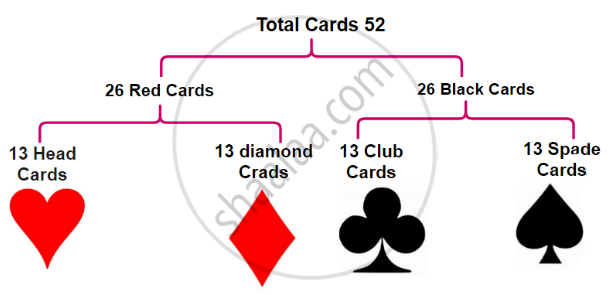# Basic Ideas of Probability:

#### 1. Random Experiment:

• The experiment in which all possible results are known in advance but none of them can be predicted with certainty and there is an equal possibility for each result is known as a ‘Random experiment’.

• For example, Tossing a coin, throwing a die, picking a card from a set of cards bearing numbers from 1 to 50, picking a card from a pack of well-shuffled playing cards, etc.

• We know all possible results of the above experiment in advance but none of them can be predicted with certainty and there is an equal possibility for each result.

#### 2. Experiment:

• An operation which can produce some well-defined outcome is called an experiment.
• In a random experiment of tossing a coin – there is only two outcomes. Head (H) or Tail (T).

#### 3. Outcome:

• Outcomes of an experiment are equally likely if each has the same chance of occurring.

• Result of a random experiment is known as an ‘Outcome’.

1. In a random experiment of tossing a coin – there are only two outcomes. Head (H) or Tail (T)
2. In a random experiment of throwing a die, there are 6 outcomes, according to the number of dots on the six faces of the die. 1 or 2 or 3 or 4 or 5 or 6.
3. A card is drawn randomly from a pack of well-shuffled playing cards.
There are 52 cards in a pack as shown below.
In a pack of playing cards, there are 4 sets, namely heart, diamond, club and spade. In each set, there are 13 cards as King, Queen, Jack, 10, 9, 8, 7, 6, 5, 4,3, 2 and Ace. King, Queen and Jack are known as face cards. In each pack of cards, there are 4 cards of the king, 4 cards of Queen and 4 cards of Jack. Thus total face cards are 12.## 4. Equally Likely Outcomes:

• A given number of outcomes are said to be equally likely if none of them occurs in preference to others.

• We assume that objects used for random experiments are fair or unbiased.

• If a die is thrown, any of the numbers from 1, 2, 3, 4, 5, 6 may appear on the upper face. It means that each number is equally likely to occur. However, if a die is so formed that a particular face comes up most often, then that die is biased. In this case, the outcomes are not likely to occur equally.

#### 5. Trial:

• A trial is an action which results in one or several outcomes.
• A trial is a single performance of the well-defined experiments, such as the flipping of a coin, the generation of a random number etc.

#### 6. Sample Space:

• The set of all possible outcomes of a random experiment is called the sample space.

• In an experiment or any random trial, when we make a set of all the results or outcomes that are possible in that experiment or trial, that set is said to be a sample space of that particular experiment.

• It is denoted by ‘S’ or ‘Ω’ (A Greek letter 'Omega').

• Each element of sample space is called a ‘sample point’.

• The number of elements in the set ‘S’ is denoted by n(S).

• If n(S) is finite, then the sample space is said to be a finite sample space.

Following are some examples of finite sample spaces.

 Sr. No Random experiment Sample space Number of sample points in S 1. One coin is tossed. S = {H, T} n(s) = 2 2. Two coins are tossed. S = { HH, HT, TH, TT} n(s) = 4 3. Three coins are tossed. S = {HHH, HHT, HTH, THH, HTT, THT, TTH, TTT} n(s) = 8 4. A die is thrown. S = {1, 2, 3, 4, 5, 6} n(s) = 6 5. Two dice are thrown. S = {(1, 1), (1, 2), (1, 3), (1, 4), (1, 5), (1, 6), (2, 1), (2, 2), (2, 3), (2, 4), (2, 5), (2, 6), (3, 1), (3, 2), (3, 3), (3, 4), (3, 5), (3, 6), (4, 1), (4, 2), (4, 3), (4, 4), (4, 5), (4, 6), (5, 1), (5, 2), (5, 3), (5, 4), (5, 5), (5, 6), (6, 1), (6, 2), (6, 3), (6, 4), (6, 5), (6, 6)} n(s) = 36 6. A card is drawn from a pack bearing numbers from 1 to 25 S = {1, 2, 3, 4,..................., 25} n(s) = 25 7. A card is drawn from a well-shuffled pack of 52 playing cards. Diamond: Ace, 2, 3, 4, 5, 6, 7, 8, 9, 10, Jack, Queen, King Spade: Ace, 2, 3, 4, 5, 6, 7, 8, 9, 10, Jack, Queen, King Heart: Ace, 2, 3, 4, 5, 6, 7, 8, 9, 10, Jack, Queen, King Club: Ace, 2, 3, 4, 5, 6, 7, 8, 9, 10, Jack, Queen, King n(s) = 52
If you would like to contribute notes or other learning material, please submit them using the button below.

### Shaalaa.com

What Is Probability? [00:16:32]
S
0%